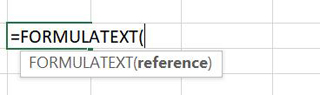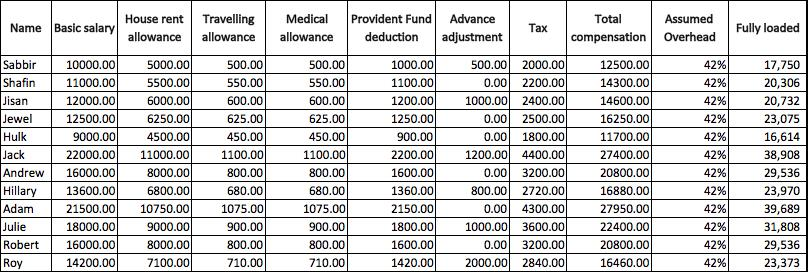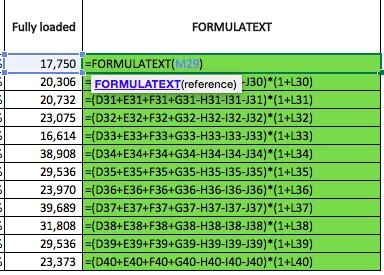FORMULA TEXT function in Excel
Wednesday, Jun 24# How to Use the FORMULA TEXT function in Excel

The Formula TEXT function in Excel is used to return a formula as text. If you have got a string of formula that is used as an equation within a cell, you can use the formula text formula and it will actually write out that formula so you can visualize it and then repeat.

Formula explanation:

• Reference: The cell which contains the formula for which we want to retrieve the text.

Example:

A Financial Analyst has obtained a compensation sheet from the HR department. He wants to print out the document for an upcoming management meeting and be able to easily explain how the fully-loaded salary is calculated for financial modeling purposes in the company’s budget.Solution:

The Financial Analyst can use the formula text formula and place the formula to the right side of the table. Then, when the document is printed, he will be able to reference it during the meeting and explain how the fully loaded compensation was calculated.Formula Implementation:Here, the net salary amounts are used as a reference.

Overall, this is a very useful formula if you want to portray the data behind a formula in a text written format, particularly if you want to print it out or just make reference to it within a sheet.

For instance, if you are sending it off to someone for review, audit, or management review and you just want to call out and show them how something is being calculated without them having to drill down into a cell.

I hope that helps. Please leave a comment below with any questions or suggestions. For more in-depth Excel training, checkout our Ultimate Excel Training Course here. Thank you!

Article RatingInline Feedbacks